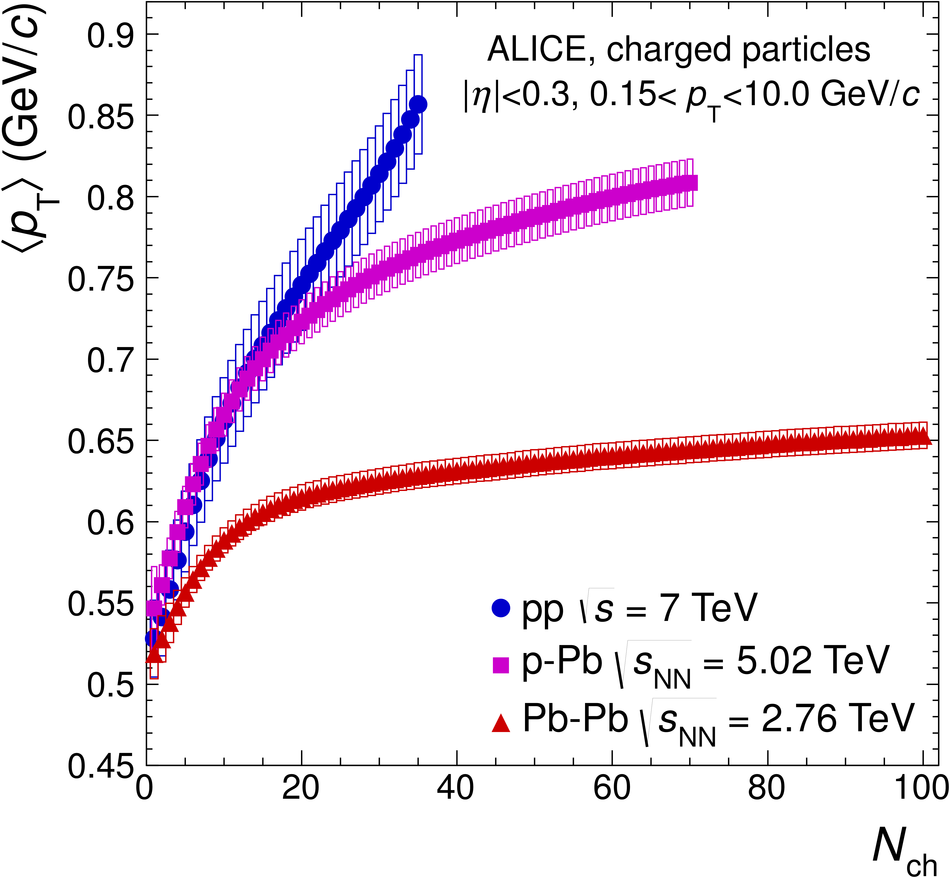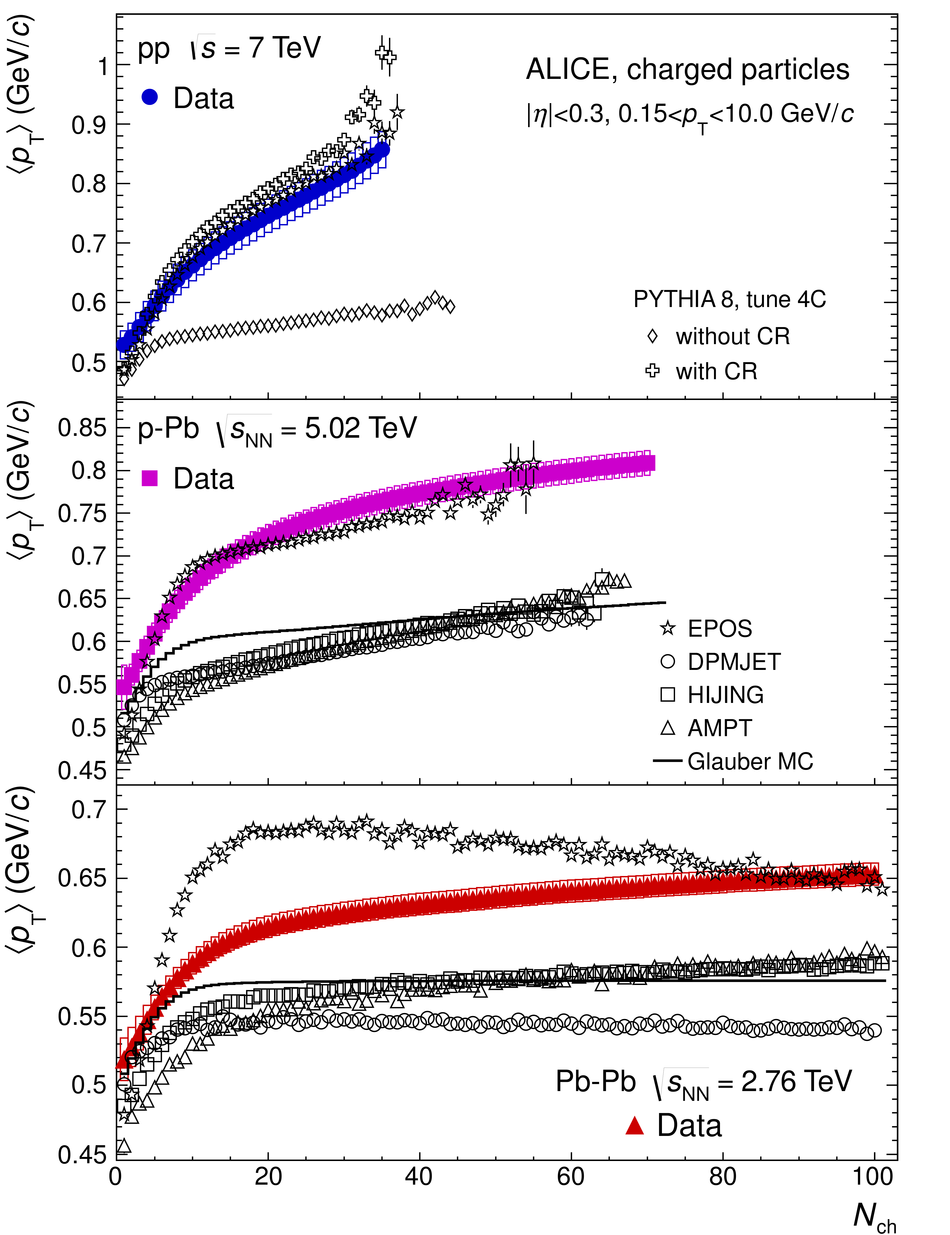# Multiplicity dependence of the average transverse momentum in pp, p-Pb, and Pb-Pb collisions at the LHC

The average transverse momentum $\langle p_{\rm T}\rangle$ versus the charged-particle multiplicity $N_{\rm ch}$ was measured in p-Pb collisions at a collision energy per nucleon-nucleon pair $\sqrt{s_{\rm NN}}=5.02$ TeV and in pp collisions at collision energies of $\sqrt{s}=0.9$, 2.76, and 7 TeV in the kinematic range $0.15<~p_{\rm T}<~10.0$ GeV/$c$ and $|\eta|<~0.3$ with the ALICE apparatus at the LHC. These data are compared to results in Pb-Pb collisions at $\sqrt{s_{\rm NN}}=2.76$ TeV at similar charged-particle multiplicities. In pp and p-Pb collisions, a strong increase of $\langle p_{\rm T}\rangle$ with $N_{\rm ch}$ is observed, which is much stronger than that measured in Pb-Pb collisions. For pp collisions, this could be attributed, within a model of hadronizing strings, to multiple-parton interactions and to a final-state color reconnection mechanism. The data in p-Pb and Pb-Pb collisions cannot be described by an incoherent superposition of nucleon-nucleon collisions and pose a challenge to most of the event generators.

Figures

## Figure 1

 Average transverse momentum $\mpt$ in the range $0.15< \pt< 10.0$ GeV/$c$ as a function of charged-particle multiplicity $\nch$ in pp collisions at $\sqrt{s}=0.9$, 2.76, and 7 TeV, for $|\eta|< 0.3$. The boxes represent the systematic uncertainties on $\mpt$. The statistical errors are negligible.## Figure 2

 Average transverse momentum $\mpt$ versus charged-particle multiplicity $\nch$ in pp, p-Pb, and Pb-Pb collisions for $|\eta|< 0.3$. The boxes represent the systematic uncertainties on $\mpt$. The statistical errors are negligible.## Figure 3

 Average transverse momentum $\mpt$ as a function of charged-particle multiplicity $\nch$ measured in pp (top panel), p-Pb (middle panel), and PbPb (lower panel) collisions in comparison to model calculations. The data are compared to calculations with the DPMJET, HIJING, AMPT, and EPOS Monte Carlo event generators. For pp collisions, calculations with PYTHIA 8 with tune 4C are shown with and without the color reconnection (CR) mechanism. The lines show calculations in a Glauber Monte Carlo approach (see text).## Figure 4

 Average transverse momentum $\mpt$ for $0< \pt< 10.0$ GeV/$c$ as a function of the scaled charged-particle multiplicity in p-Pb and pp collisions for $|\eta|< 0.3$. The boxes represent the systematic uncertainties on $\mpt$. The statistical errors are negligible.